Download Presentation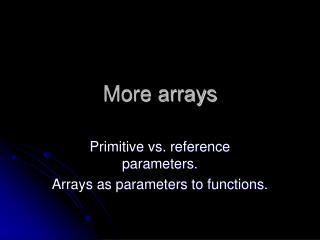More arrays

# More arrays - PowerPoint PPT Presentation

More arrays. Primitive vs. reference parameters. Arrays as parameters to functions. Recall from our room reservation problem how we initialized our hotel array. final int N = 100; //# of rooms //note: there are N rooms which are subscripted 0..N-1. boolean rooms[] = new boolean [ N ];I am the owner, or an agent authorized to act on behalf of the owner, of the copyrighted work described.
Download Presentation## More arrays

An Image/Link below is provided (as is) to download presentation

Download Policy: Content on the Website is provided to you AS IS for your information and personal use and may not be sold / licensed / shared on other websites without getting consent from its author.While downloading, if for some reason you are not able to download a presentation, the publisher may have deleted the file from their server.

- - - - - - - - - - - - - - - - - - - - - - - - - - E N D - - - - - - - - - - - - - - - - - - - - - - - - - -
Presentation Transcript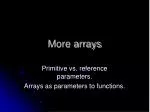### More arrays

Primitive vs. reference parameters.

Arrays as parameters to functions.Recall from our room reservation problem how we initialized our hotel array.

final int N = 100; //# of rooms

//note: there are N rooms which are subscripted 0..N-1.

boolean rooms[] = new boolean[ N ];

//init all rooms to unoccupied

for (inti=0; i<N; i++) {

rooms[i] = false;

}

Can we put this code in a function that we call from our main function?

Can we use arrays as function parameters?Warning!

Technical

definitions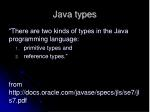Java types

“There are two kinds of types in the Java programming language:

• primitive types and
• reference types.”

from http://docs.oracle.com/javase/specs/jls/se7/jls7.pdf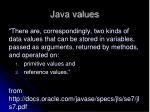Java values

“There are, correspondingly, two kinds of data values that can be stored in variables, passed as arguments, returned by methods, and operated on:

• primitive values and
• reference values.”

from http://docs.oracle.com/javase/specs/jls/se7/jls7.pdfPrimitive types
• boolean
• byte
• short
• int
• long
• char
• float
• double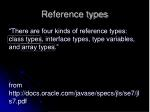Reference types

“There are four kinds of reference types: class types, interface types, type variables, and array types.”

from http://docs.oracle.com/javase/specs/jls/se7/jls7.pdf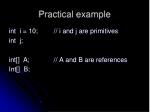Practical example

inti = 10; // i and j are primitives

int j;

int[] A; // A and B are references

Int[] B;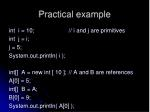Practical example

inti = 10; // i and j are primitives

int j = i;

j = 5;

System.out.println( i );

int[] A = new int [ 10 ]; // A and B are references

A = 5;

int[] B = A;

B = 9;

System.out.println( A );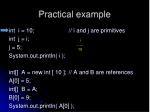Practical example

inti = 10; // i and j are primitives

int j = i;

j = 5;

System.out.println( i );

int[] A = new int [ 10 ]; // A and B are references

A = 5;

int[] B = A;

B = 9;

System.out.println( A );

i

-----

10Practical example

inti = 10; // i and j are primitives

int j = i;

j = 5;

System.out.println( i );

int[] A = new int [ 10 ]; // A and B are references

A = 5;

int[] B = A;

B = 9;

System.out.println( A );

j

-----

10

i

-----

10Practical example

inti = 10; // i and j are primitives

int j = i;

j = 5;

System.out.println( i );

int[] A = new int [ 10 ]; // A and B are references

A = 5;

int[] B = A;

B = 9;

System.out.println( A );

j

-----

10 5

i

-----

10

XPractical example

inti = 10; // i and j are primitives

int j = i;

j = 5;

System.out.println( i ); 10

int[] A = new int [ 10 ]; // A and B are references

A = 5;

int[] B = A;

B = 9;

System.out.println( A );

j

-----

10 5

i

-----

10

XPractical example

inti = 10; // i and j are primitives

int j = i;

j = 5;

System.out.println( i ); 10

int[] A = new int [ 10 ]; // A and B are references

A = 5;

int[] B = A;

B = 9;

System.out.println( A );

j

-----

10 5

i

-----

10

X

A

-----

.

-------

-------

-------

-------Practical example

inti = 10; // i and j are primitives

int j = i;

j = 5;

System.out.println( i ); 10

int[] A = new int [ 10 ]; // A and B are references

A = 5;

int[] B = A;

B = 9;

System.out.println( A );

j

-----

10 5

i

-----

10

X

A

-----

.

-------

5

-------

-------

-------Practical example

inti = 10; // i and j are primitives

int j = i;

j = 5;

System.out.println( i ); 10

int[] A = new int [ 10 ]; // A and B are references

A = 5;

int[] B = A;

B = 9;

System.out.println( A );

j

-----

10 5

i

-----

10

X

B

-----

.

A

-----

.

-------

5

-------

-------

-------Practical example

inti = 10; // i and j are primitives

int j = i;

j = 5;

System.out.println( i ); 10

int[] A = new int [ 10 ]; // A and B are references

A = 5;

int[] B = A;

B = 9;

System.out.println( A );

j

-----

10 5

i

-----

10

X

B

-----

.

A

-----

.

-------

5 9

-------

-------

-------

XPractical example

inti = 10; // i and j are primitives

int j = i;

j = 5;

System.out.println( i ); 10

int[] A = new int [ 10 ]; // A and B are references

A = 5;

int[] B = A;

B = 9;

System.out.println( A ); 9

j

-----

10 5

i

-----

10

X

B

-----

.

A

-----

.

-------

5 9

-------

-------

-------

XRecall from our room reservation problem how we initialized our hotel array.

final int N = 100; //# of rooms

boolean rooms[] = new boolean[ N ];

//init all rooms to unoccupied

for (inti=0; i<N; i++) {

rooms[i] = false;

}

Can we put this code in a function that we call from our main function? Yes!

Can we use arrays as function parameters? Yes!Our hotel reservation system.

class Hotel {

static void initializeHotel ( boolean occupied[] ) {

//init all rooms to unoccupied

for (int i=0; i<occupied.length; i++) {

occupied[i] = false;

}

}

public static void main ( String args[] ) {

//How do we use this new function from main?

}

}Our hotel reservation system.

class Hotel {

static void initializeHotel ( boolean occupied[] ) {

//init all rooms to unoccupied

for (inti=0; i<occupied.length; i++) {

occupied[i] = false;

}

}

public static void main ( String args[] ) {

final int N = 100; //# of rooms

//var to indicate occupied/unoccupied rooms

boolean rooms[] = new boolean[ N ];

//How do we use initializeHotel from main?

}

}Our hotel reservation system.

class Hotel {

static void initializeHotel ( boolean occupied[] ) {

//init all rooms to unoccupied

for (inti=0; i<occupied.length; i++) {

occupied[i] = false;

}

}

public static void main ( String args[] ) {

final int N = 100; //# of rooms

//var to indicate occupied/unoccupied rooms

boolean rooms[] = new boolean[ N ];

initializeHotel( rooms );

}

}Our hotel reservation system.

class Hotel {

//init our hotel to all empty

static void initializeHotel ( boolean occupied[] ) {

//init all rooms to unoccupied

for (inti=0; i<occupied.length; i++) {

occupied[i] = false;

}

}

public static void main ( String args[] ) {

final int N = 100; //# of rooms

//var to indicate occupied/unoccupied rooms

boolean rooms[] = new boolean[ N ];

initializeHotel( rooms );

}

}

This is an example of a parameter with a reference value. Changes made in initializeHotel to occupied array entries change rooms array entries in main.Our hotel reservation system.
• How about another function to find the first free (available/unoccupied) room?
• It should return the room # of the first available room.
• If no rooms are available, it should return -1.
• We will also specify a range of room numbers to check (we won’t always check the entire hotel).So far we have…

class Hotel {

static void initializeHotel ( boolean occupied[] ) {

}

public static void main ( String args[] ) {

}

}So far we have…

class Hotel {

static void initializeHotel ( boolean occupied[] ) {

}

static intfirstFree ( int first, int last, boolean occupied[] ) {

}

public static void main ( String args[] ) {

}

}Our hotel reservation system:first free room

//This function returns the number of the first available

// in the range of [first,last] inclusive.

// If no room can be found, -1 is returned.

static intfirstFree ( int first, int last, boolean occupied[] ) {

int found = -1;

for (int i=first; i<=last; i++) {

//find the first room that is not occupied

if ( !occupied[i] ) {

found = i; //remember the free room #

break; //terminate the loop

}

}

return found;

}### How can we use this new function in main to check is a room between # 10 and # 20 is available?How can we use this new function in main to check is a room between # 10 and # 20 is available?

public static void main ( String args[] ) {

final int N = 100; //# of rooms

//var to indicate occupied/unoccupied rooms

boolean rooms[] = new boolean[ N ];

initializeHotel( rooms );

//check for a room between 10 and 20

intrm = firstFree( 10, 20, rooms );

System.out.println( "room # " + rm + " is available" );

}

In the call to firstFree, 10 and 20 are primitive values, and rooms is a reference value.### How can we use this new function in main to check our entire hotel for the first free room?//check for a room in the entire hotel

int r = firstFree( 0, N-1, rooms );

System.out.println( "room # " + r

+ " is available" );

In this call to firstFree, 0 and N-1 are primitive values, and rooms is a reference value.Passed by value vs. passed by reference
• So what’s the difference?
• For a primitive value, any changes to the variable in the function DO NOT affect the caller.
• For a reference value, any changes in the function to the data to which the reference refers DO affect the caller.Passed by value vs. passed by reference example.

static void test ( int A, int B[] ) {

A = A + 12;

B = B + 12;

}

In main:

int A = 5;

int D[] = new int ;

D = 5;

D = 5;

test( A, D );

System.out.println( "A is " + A + “, D is " + D );

What’s the output?

Remember, A is a primitive value, and D is a reference value.Passed by value vs. passed by reference example.

static void test ( int A, int B[] ) {

A = A + 12;

B = B + 12;

}

In main:

int A = 5;

int D[] = new int ;

D = 5;

D = 5;

test( A, D );

System.out.println( "A is " + A + “, D is " + D );

What’s the output?

A is 5, D is 17

test made changes to both A and B but since A is a primitive value, those changes don’t affect A in main.

Since B is a reference value, the changes made by test to B do affect D in main.Back to our hotel reservation system…
• Write a function that, given a room number and our rooms array, indicates that that room is occupied.So far we have…

class Hotel {

static void initializeHotel ( boolean occupied[] ) {

}

static intfirstFree ( int first, int last, boolean occupied[] ) {

}

public static void main ( String args[] ) {

}

}So far we have…

class Hotel {

static void bookARoom ( intrm, boolean rooms[] ) {

}

static void initializeHotel ( boolean occupied[] ) {

}

static intfirstFree ( int first, int last, boolean occupied[] ) {

}

public static void main ( String args[] ) {

}

}Back to our hotel reservation system…
• Write a function that, given a room number and our rooms array, indicates that that room is occupied.

static void bookARoom ( intrm, boolean rooms[] )

{

… //what do we need to do here?

}Back to our hotel reservation system…
• Write a function that, given a room number and our rooms array, indicates that that room is occupied.

static void bookARoom ( int rm, boolean rooms[] )

{

rooms[ rm ] = true;

}Back to our hotel reservation system…

//given a room number and our rooms array,

// indicate that that room is occupied.

static void bookARoom ( int rm, boolean rooms[] )

{

//it would be wise to check rm here first to determine if

// it is between 1..# of rooms.

rooms[ rm ] = true;

}

In main:

bookARoom( 1, rooms );

bookARoom( 2, rooms );

bookARoom( 10, rooms );More hotel reservation system…
• Wouldn’t it be useful to know how many rooms are occupied in our hotel?
• We can write function to do that!So far we have…

class Hotel {

static void bookARoom ( intrm, boolean rooms[] ) {

}

static void initializeHotel ( boolean occupied[] ) {

}

static intfirstFree ( int first, int last, boolean occupied[] ) {

}

public static void main ( String args[] ) {

}

static intoccupiedCount ( boolean rooms[] ) {

}

}More hotel reservation system…

//function that determines how many rooms

// are occupied in our hotel

static intoccupiedCount ( boolean rooms[] )

{

int count = 0;

for (inti=0; i<rooms.length; i++) {

//check here needed.

}

}More hotel reservation system…

//function that determines how many rooms

// are occupied in our hotel

static intoccupiedCount ( boolean rooms[] )

{

int count = 0;

for (inti=0; i<rooms.length; i++) {

if ( rooms[i] )

++count;

}

return count;

}More hotel reservation system…

//function that determines how many rooms

// are occupied in our hotel

static intoccupiedCount ( boolean rooms[] )

{

int count = 0;

for (inti=0; i<rooms.length; i++) {

if ( rooms[i] )

++count;

}

return count;

}

Now, how can we use this new function from main?Room in use.

In main:

bookARoom( 1, rooms );

bookARoom( 2, rooms );

bookARoom( 10, rooms );

System.out.println( occupiedCount(rooms)

+ " in use." );Hotel reservation system.
• Other useful functions include:
• Write a function that returns the percentage of free rooms in the hotel. (Your function should call occupiedCount.)Hotel reservation system.
• Other useful functions include:
• Write a function to allow someone to check out. Given a room number, this function should indicate that the room is no longer occupied.Hotel reservation system.
• Other useful functions include:
• Write a function to find a block (i.e., contiguous) of 3 rooms.
• Return the room # of the first room in the block.
• If no such block is available, return -1.Hotel reservation system.
• Other useful functions include:
• Write a function called findARoom that finds an available room.
• But we don’t want to simply use firstFree because we’ll wear out some rooms in our hotel and not use other rooms at all.
• So findARoom should generate a random number and see if that room is free. If it’s not free, findARoom can then use firstFree.Java types

“There are two kinds of types in the Java programming language:

• primitive types and
• reference types.”

from http://docs.oracle.com/javase/specs/jls/se7/jls7.pdfJava values

“There are, correspondingly, two kinds of data values that can be stored in variables, passed as arguments, returned by methods, and operated on:

• primitive values and
• reference values.”

from http://docs.oracle.com/javase/specs/jls/se7/jls7.pdfFunction parameters, and primitive and reference values.
• Changes to primitives do not affect the calling function.
• Changes to references (more specifically, changes to what the reference refers) do affect the calling function.